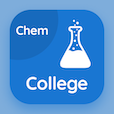Colleges Online Courses

College Chemistry MCQs

College Chemistry MCQ PDF - Topics

# Ideal Gas Constant MCQ Quiz Online

Practice Ideal Gas Constant Multiple Choice Questions (MCQ), Ideal Gas Constant quiz answers PDF to learn college chemistry online course for college chemistry classes. Gases Multiple Choice Questions and Answers (MCQs), Ideal Gas Constant quiz questions for GRE practice test. "Ideal Gas Constant MCQ" PDF Book: plasma state, avogadro's law, liquefaction of gases, diffusion and effusion test prep for SAT test.

"According to standard units, the value of R is" MCQ PDF: ideal gas constant with choices 0.0765, 0.082, 0.074, and 0.087 for GRE practice test. Learn ideal gas constant quiz questions for merit scholarship test and certificate programs for online college bachelor degree.

## MCQs on Ideal Gas Constant Quiz

MCQ: According to standard units, the value of R is

0.0765
0.082
0.074
0.087

MCQ: One mole of gas at ideal conditions have a volume of

22.414dm cube
24.14 dm3
37.14dm cube
44.14 dm cube

MCQ: Standard temperature is considered to be as

234.17K
244K
279.14K
273.16K

MCQ: R becomes 8.3143 when energy is taken in

Newton per meter square
torr
calories
joule

MCQ: One meter cube is equal to

100 dm cube
1000 dm cube
10 dm cube
50 dm cube

### More Topics from College Chemistry Course